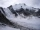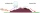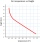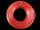# Temperature + functions - math problems

#### Number of problems found: 5

• The temperature 5The temperature at 4pm was 53° Fahrenheit the temperature dropped 3° each hour from 4pm to 9pm which equation below accurately represents the temperature at 9pm
• ImagineImagine that a unit of air at a temperature of 25°C rises up a mountain range that is 3,000 meters high on the windward side and which descends to 1,200 meters on the leeward side, assuming that the air will remain dry what will its temperature when it cr
• Air thermalImagine that a unit of air rises at 3000 meters high, if temperature decreases 6 degrees celcius for every 1000 meter, what will be its temperature at 1400 meters, 2000 meters, 2500 meters and when it reaches the 3000 meter elevation. Starting temperature
• Electrics - conductorThe wire is 106 meters long at 0 °C and at every temperature increase of 1 °C the length increases by 0.15 mm per 1 m length of wire. Determine a function which represents the overall length of the wire as a function of temperature. What is the length of
• Degrees Fahrenheit? The equation above shows how temperature F, measured in degrees Fahrenheit, relates to a temperature C, measured in degrees Celsius. Based on the equation, which of the following must be true? I. A temperature increase of 1 degree Fahrenheit is equivale

We apologize, but in this category are not a lot of examples.
Do you have an interesting mathematical word problem that you can't solve it? Submit a math problem, and we can try to solve it.

We will send a solution to your e-mail address. Solved examples are also published here. Please enter the e-mail correctly and check whether you don't have a full mailbox.

Please do not submit problems from current active competitions such as Mathematical Olympiad, correspondence seminars etc...